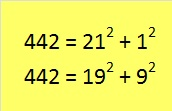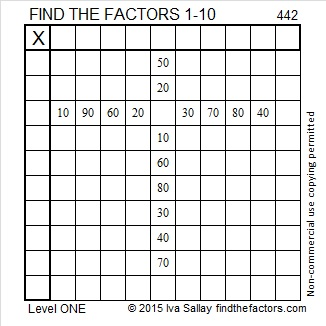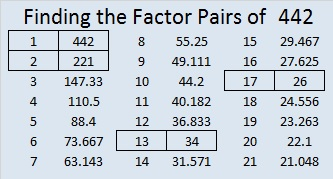# 442 and Level 1

442 is the sum of two square numbers two different ways:Even number 442 is also the sum of consecutive primes. Will the number of consecutive primes be even or odd? If you figure out what those prime numbers are, look in the comments to see if you were right.Print the puzzles or type the factors on this excel file: 10 Factors 2015-03-30

Here is the factoring information for 442:

• 442 is a composite number.
• Prime factorization: 442 = 2 x 13 x 17
• The exponents in the prime factorization are 1, 1, and 1. Adding one to each and multiplying we get (1 + 1)(1 + 1)(1 + 1) = 2 x 2 x 2 = 8. Therefore 442 has exactly 8 factors.
• Factors of 442: 1, 2, 13, 17, 26, 34, 221, 442
• Factor pairs: 442 = 1 x 442, 2 x 221, 13 x 34, or 17 x 26
• 442 has no square factors that allow its square root to be simplified. √442 ≈ 21.0238442 is the hypotenuse of four Pythagorean triples:

• 170-408-442 which is [5-12-13] times 34
• 208-390-442 which is [8-15-17] times 26
• 280-342-442 which is [140-171-221] times 2
• 42-440-442 which is [21-220-221] times 2## 3 thoughts on “442 and Level 1”

1.ivasallay

Alan Parr wrote the following in the comments on post number 463:

442’s OK; it’s 41+43+47+53+59+61+67+71

2.abyssbrain

41 + 43 + 47 + 53 + 59 + 61 + 67 + 71 = 442

It took me a while to find this 🙂

•ivasallay

Great minds think alike! Congratulations to both of you.

This site uses Akismet to reduce spam. Learn how your comment data is processed.